﻿ Functionally Graded Sandwich Circular Plate of Non-Uniform Varying Thickness with Homogenous Core Resting on Elastic Foundation: Investigation on Bending via Differential Quadrature MethodPublications are Open
Access in this journal
Article Versions
Export Article
Cite this article
• Normal Style
• MLA Style
• APA Style
• Chicago Style
Research Article
Open Access Peer-reviewed

### Functionally Graded Sandwich Circular Plate of Non-Uniform Varying Thickness with Homogenous Core Resting on Elastic Foundation: Investigation on Bending via Differential Quadrature Method

Fatemeh Farhatnia , Reza Saadat, Soheil Oveissi
American Journal of Mechanical Engineering. 2019, 7(2), 68-78. DOI: 10.12691/ajme-7-2-3
Received January 11, 2019; Revised February 25, 2019; Accepted April 18, 2019

### Abstract

This paper illustrates the effectiveness of two functionally graded (FG) layers on a homogenous circular plate for bending analysis. The classical plate theory (CPT) serves as the basis of the analysis. Differential quadrature method (DQM) as semi-analytical method is employed to solve the governing equations. The material properties is varied to obey power-law in terms of the plate thickness direction. The plate is subjected to uniform transverse loading and resting on Winkler elastic foundation. In this study, the effect of the different profile of the plate thickness, elastic foundation coefficient, the volume fraction FG index, and effect of the boundary conditions, namely, simply supported and clamped edge on static response are demonstrated. The results are compared with finite element method and published literature that observed to be in accordance with each other.

### 1. Introduction

In the modern industrial societies, materials play an essential role; for instance, advanced composite materials offer numerous superior properties to metallic materials, such as high specific strength and high specific stiffness that its consequence is the extensive use of laminated composite materials. Functionally Graded Materials (FGMs) are advanced engineered materials whereby material composition and properties vary spatially in macroscopic length scales that are fabricated by the specialized manufacturing process 1. FGMs possess a continuous variation of material properties in one or more directions and are typically made from a mixture of ceramics and metals with the variation of the volume fraction according to a power law through thickness 2, 3. Nowadays, FGMs are alternative materials widely used in aerospace, civil and automotive engineering, as well as biomechanical applications. Furthermore, sandwich structures have wide applications in many industries such as aerospace and also widely in aircraft, space vehicles, ships, bridges, nuclear and solar plants. In general, the sandwich structures of functionally graded materials are composed of two FG layers, which are not necessarily similar to each other. The main characteristic of this kind of materials, which makes them discern from the conventional materials is resistant to environment high temperature. Nevertheless, a sudden change of mechanical properties of materials between the layers gives rise to an out-plane tension 4. In order to overcome interface problems between faces and core as apparent in conventional sandwich structures, FG sandwich plates have recently been used. Consequently, the mechanical behaviors of this kind of plates have been examined by some authors with analytical and numerical approaches 5, 6, 7, 8, 9, 10, 11, 12. Praveen and Reddy 13 investigated the static and dynamic response of functionally graded ceramic–metal plates in the thermal environment, using finite element method that accounts for the transverse shear strains, rotary inertia and moderately large rotations based on the Von-Karman nonlinearity. Houari et al. 14 investigated thermoelastic bending of the functionally graded sandwich plate using the theory, in which the transverse displacement is divided into bending, shear and thickness stretching parts. Huanga et al 15 revealed that in the classical analysis of bending of a circular plate with simply supported edges when subjected to transverse loading, the effect of the radial component of the reaction force is often neglected. They analyzed the effect of the radial component of the reaction force on the bending of thin circular plates using the Kirchhoff plate theory. Chen and Fang 16 analyzed the deformation and stability of a circular plate under its own weight, supported by a flexible concentric ring, and found out a stable non-axisymmetric warping of a heavy circular plate.

Also, Influence of elastic foundation on mechanical behavior of FG plates is found as a subject of interest by some researchers. Alipour et al. 17 examined the free vibration of functionally graded circular sandwich plate on Winkler elastic foundation based on the zigzag theory. Mantari et al. 18 studied the buckling, bending and free vibration analysis of different layer thickness ratios functionally graded sandwich plates using a new optimized hyperbolic displacement under Carrera’s Unified formulation. Carrera et al. 19 proposed a new plate finite element for the analysis of composite and sandwich plates using the node-variable plate theory assumptions. The exact solution of the stability analysis of the circular plates with foundation and elastic restrained edge against translation and rotation is reported by et al. 20. Farhatnia et al. 21 investigated on thermal buckling behavior of circular FG orthotropic plate resting on Pasternak elastic foundation via differential transform method (DTM).

Nowadays, the influence of variable thickness on dynamic and static response of FG structures has been received the attention by some researchers. This is due to the application in the design and construction of machinery parts. Exact vibration analysis of an FGM annular plate of variable thickness is performed using the first-order shear deformation plate theory using the exact element method by Efraim and Eisenberger 22. Naei et al. 23 studied the buckling analysis of radially-loaded FGM circular plates with variable thickness using energy method based on Love–Kirchhoff hypothesis and Sander’s non-linear strain–displacement relation for thin plates. Xiang and Yang 24 presented the free and forced vibration of a laminated functionally graded Timoshenko beam of variable thickness, which consists of a homogeneous substrate and two inhomogeneous functionally graded layers. They employed differential quadrature method by using of Lagrange interpolation polynomials as a numerical solution tool to solve the governing differential equations. Xu and Zhou 25 investigated the stress and displacement distributions of continuously varying thickness functionally graded rectangular plates with simply supported at four edges via the finite element method. Farhatnia and Golshah 26 investigated on buckling of orthotropic circular and annular plates of continuously variable thickness via optimized Ritz method. Vivio and Vullo 27 studied axisymmetric bending of circular plates with variable thickness, subjected to symmetrical loading. They proposed a new analytical method to determine elastic stresses and deformations on the basis of the two independent integrals of the hypergeometric differential equation.

The present work is the first attempt to study the bending behavior of the three-layer FGM sandwich circular plate of varying thickness resting on the Winkler elastic foundation under uniform distributed transverse loading. The plate is composed of two heterogeneous isotropic materials (FG) at the upper and lower layers, whereas the core is homogenous. The Young modulus of the two FG layers are considered to be varying according to a power-law distribution in terms of the volume fraction of the constituent. The governing equation is solved using the differential quadrature method as semi-analytical approach. The numerical results of three-layer FGM sandwich circular plate with simply supported and clamped boundary conditions are obtained with the computer programming using MAPLE and MATLAB, as well as the simulating in ABAQUS software for comparison of the results.

### 2. Governing Equations

2.1. Statement of the Problem

Consider a three-layered circular sandwich plate of radius a, including a homogenous core with variable thickness and two non-homogenous isotropic layers with uniform thickness, which its properties are varied along the thickness direction, continuously and smoothly. As demonstrated in Figure 1, the plate is under a uniform distributed loading p and is embedded on a Winkler elastic medium with elastic coefficient kw. Let the plate be referred to a cylindrical polar coordinate system (r θ, z). z=0 being the middle plane of the plate. The top and bottom surfaces are z =-h/2 and z=h/2, respectively.

• Figure 1. The three-layered circular sandwich plate with variable thickness resting on Winkler elastic foundation

The classical plate theory based on Kirchhoff hypothesis is employed to derive the governing equation of bending with assumption of non-uniformity of thickness. This equation is derived based on the neutral plane, which is not coincident on the mid-plane for the non-homogenous material. These equations are derived based on the neutral plane, which is not coincident on the mid-plane for the non-homogenous material. In the classical theory of plates, the displacement field in polar coordinate is defined as follows 28: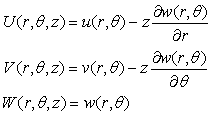(1)

where u, v, and w are the displacements of the plate in the plane z=0. Using Eq. (1), one obtains the following strain-displacement as: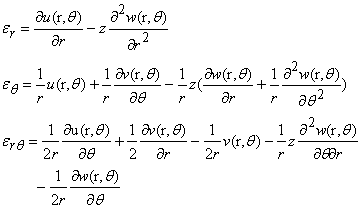(2)

where εr, εθ and ε are the radial, tangential and shear strains, respectively. Herein, Due to symmetry, tangential displacement and the differentiation with respect to circumferential direction equals to zero, ∂/∂θ=0. Moreover, in the plane-stress condition, the stress relations for FG circular plate, are as follows 29: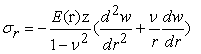(3a)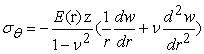(3b)

where ν is Poisson’s ratio, σr and σθ are the radial and tangential stress, respectively. E(r) is the Young’s modulus that is defined as follows: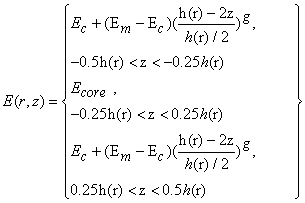(4)

where Em, Ec and Ecore are the elasticity modulus of the metal, ceramic and core, respectively, and g is the volume fraction exponent. In addition, the flexural rigidity of three-layered sandwich plate is determined, as: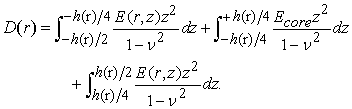(5)

Therefore, neglecting the in-plane stress resultants, the stress couples are obtained as follows 28: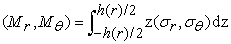(6a)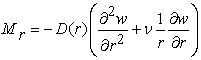(6b)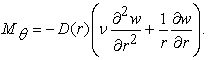(6c)

The governing equations for the static response analysis of sandwich thin circular plate can be obtained by using the principle of Hamilton, which states 30: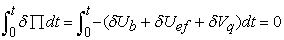(7)

where δ stands for variation operator,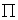is the plate total potential energy, and Ub, Uef, Vq represent the bending strain energy of the plate, the elastic foundation energy, and in this study the potential of external work done by transverse uniform loading on the plate, respectively.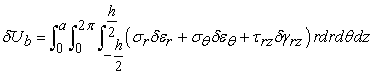(8a)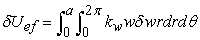(8b)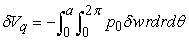(8c)

Where kw denotes coefficient of Winkler elastic foundation, that is the most commonly used mechanical model for the elastic foundation. Winkler elastic foundation is simulated using of a set of linear elastic springs which work independently. In addition, it was known as one-parameter model 31. Upon substituting stress relation (3) and strain relation (2) in form of symmetric condition into (8a) and by neglecting influence of shear deformation, carrying out some mathematical simplification, the governing equation polar coordinates, is obtained as: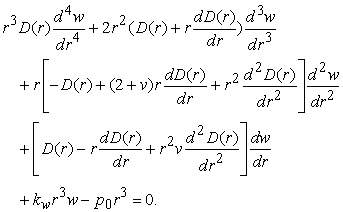(9)
2.2. Profile of the Variable Thickness

As mentioned before, the plate thickness is non-uniform along the radial direction that its profile can be defined as follows: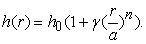(10)

in which h0 dnotes the reference thickness, n and γ are the geometrical parameters, and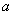is the circular plate radius. Variations of thickness profile are sketched in Figure 2 (a-c).

As shown, the thickness is constant for γ=0, and it is changed linearly, if n=1. The plate concavity is increased by increasing the value of n. It may be noted that this variations are decreased for n>5. The thickness profile concavity gets upward for γ>0, whereas gets downward for γ<0. Herein, values of n are 1, 3 and 5 are considered.

• Figure 2. The profil of the plate thickness function for h0=0.01 and (a) n=1, (b) n=3, (c) n=5

### 3. Application of the Differential Quadratic Method into governing equations

Always, Finding the exact solution for governing equations of engineering problems are not feasible; therefore with increasing the capability of personal computers, a variety of numerical methods, i.e. finite element method, boundary element method and finite differences method are available for engineering aims 32. DQM is a numerical approach for solving partial, linear and non-linear differential equations. In order to solve numerically the governing equation of the circular plate, the differential quadratic method (DQM) is utilized. To this end, the plate is discretized as Figure 3. As shown in this figure, the mesh includes n nodes in its radius direction and p nodes on the circumference of circle. Using partial differentiate of nth order and mth order of f(r,t) respectively to R and T, the following relations are yielded 33: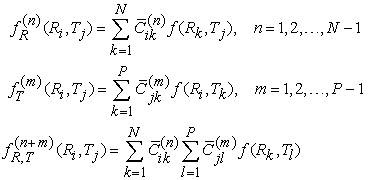(11)

in which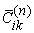and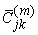are the weighting coefficients of n-th and m-th order partial derivatives of f(r,t), respectively. The weighting coefficients are defined as follows: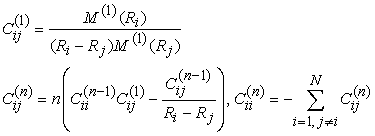(12)

where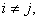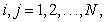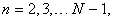and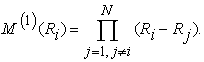• Figure 3. A type of two-dimensional meshing for DQM in polar coordinates

Furthermore, to achieve reasonably convergence and to yield better accuracy, unequally space grid points are chosen. For this reason, Chebyshev distribution technique can be employed 30: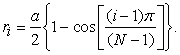(13)

To discretize the governing equation by using the relations (10), the DQM is applied as follows: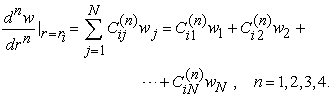(14)

The matrix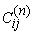is achieved for Chebyshev distribution points utilizing the Maple 8.0 software. Herein, for brevity purpose, only the discretized form of an equilibrium equation and a boundary condition are presented. Afterwards, in order to solve the governing equation, relation (12) is substituted into Eq. (7), as follow: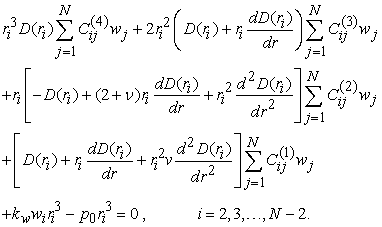(15)

Furthermore, as the same way, the radial and tangential stresses at any arbitrary grid point, Eq. (3), are written as: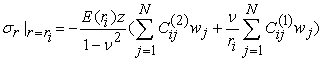(16a)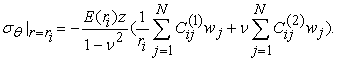(16b)
3.1. Boundary Conditions

Two boundary conditions are considered in the present paper, namely, clamped and simply-supported edges:

- Clamped edge: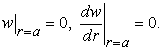(17)

- Simply-supported edge: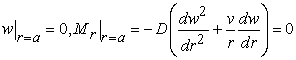(18)

-Regularity condition:

For a solid circular plate, the regularity condition is required to confirm that the solution is avoided form singularity, is defined as: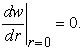(19)

To analyze the thin plate, employing differential quadrature method (DQM) arises some difficulty. This is due to existence of two boundary conditions for each grid point, whereas only one differential equation is governed 34. Bert 35 considered δ-point technique is an adequate way for overcome this inconvenience and it is quite successfully when applying for the double boundary conditions of beam and plate problems. In this technique, one boundary condition is written for boundary grid points and the other condition is imposed on the δ-point. The discretized form of supporting conditions including regularity conditions and boundary conditions are given as:

Clamped edge: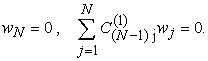(20)

Simply supported edge: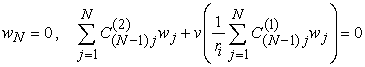(21)

Regularity condition at center of the plate: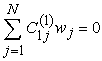(22)

In order to achieve the solution, the discretized forms of boundary and regularity conditions and governing equation should be solved simultaneously. The assembly of Equation (15) through Equation (22) yields the followings set of equations in form of matrix: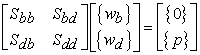(23)

where, Sdd, Sbb and Sbd are of orders (N-3)×(N-3), (3×3) and 3×(N-3), respectively. The system of linear equations (23), the domain and boundary grid points are separated and represented in vector forms, they are denoted as {d} and {b}, respectively. By matrix sub-structuring of Equation (23), we have the following two equations: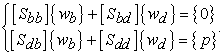(24)

From the first part of Equation (24), we obtain: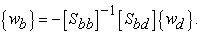(25)

Back-substituting Equation (25) into the second part of Equation (24), we get: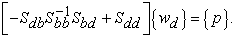(26)

By solving the linear set of equation, By taking into account the imposed supporting conditions, coding in MAPLE 8.0 software for solving governing equation in order to derive the stiffness matrix and using MATLAB 6.1 programming to solve the repetitive steps for obtaining the displacement in grid points, the numerical results are obtained in the next section.

### 4. Results and Discussion

Several examples are performed concerning bending response of FG sandwich plates with homogenous core resting on elastic foundation. The mechanical and geometrical properties utilized in this study are given in Table 1.

In addition, the core of plate is considered as a homogeneous material. In this section, Non-dimensionalized deflection and stresses given here are presented according to the following: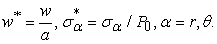4.1. Validation

In order to validate the accuracy of the results, in Table 2, the present results are compared with those available in Abbasi et al. 36 in a homogeneous plate with γ=0 (i.e. the plate thickness is constant) and various values of the Winkler modulus. In this study, in order to achieve the convergence with acceptable accuracy, N=12 and N=18 (number of grid points) are sufficient for the clamped and simply-supported plates respectively. In both cases of boundary conditions, δ=10-4. In order to validate the DQM analysis, the finite element simulation of sandwich plate with two FG coatings is carried out using ABAQUS 6.13 software and represented in Table 3 and Table 4. For modeling in ABAQUS, the plate is meshed using Defomable 3D Solid element and consists of 27448 elements. FG layers is divided into 10 equal sub-layers along the thickness direction. The material properties of each sub-layer are calculated according to power law functions given in Eq. (1). It may be noted, c and s represent the clamped and simply supported edges, respectively. The results are compared with FEM for the case of γ=0, kw=0 and variation of g in Table 3, which indicate magnitudes of the displacement of FGM circular plate center. In addition, for different values of γ and kw=0, the Table 4 lists the magnitude of deflection for circular homogeneous plate with non-uniform thickness and compared with those obtained by ABAQUS simulation.

For the lower stiffness of the plate based on FEM results, the quantities of deflection gets more than those obtained by DQM method with the relative error of less than 0.02. This relative discrepancy is justified by three-dimensional solving in ABAQUS. The comparison of radial and tangential stress between present approach and FEM are carried out and represented in Table 5 and Table 6.

4.2. Effects of Various Parameters on the Plate Deflection

In this subsection, the effects of various parameters such as n and γ (thickness characteristics), kw and g are examined on the deflection of three-layer FGM circular sandwich plate for both simply supported and clamped edge conditions. The results for the various values of these parameters are given in Table 7, Table 8 and Table 9, respectively for n=1, 3, and 5, respectively.

In Figure 4, for n=3, kw=1, and g=1, the variation of the dimensionless deflection of a three-layer FGM circular sandwich plate under the uniform distributed loading is sketched for two boundary conditions, namely, simply supported and clamped edges. Figure 4 reveals, for a constant value of n, the deflection is decreased by increasing γ. Moreover, the deflection would decrease when upgrading the restraint on the rotation at the edges. Figure 5 and Figure 6 demonstrate the deflection of plates under uniform distributed loading is strongly influenced by the elastic medium. So that, by increasing the stiffness of Winkler foundation in the range of zero to 20, the maximum deflection of the plate is decreased, prominently. Moreover, as illustrated in Figure 6, for the larger values of the volume fraction, g, leads to an increase in deflection, due to the reason that increasing g causes to decrease the plate flexural rigidity and consequently, the deformation of the plate is increased.

• Figure 4. Variations of the dimensionless deflection of FGM circular sandwich plate versus the dimensionless circular plate radius for: (a) simply supported end (b) clamped end, n=3, kw=1, g=1
• Figure 5. Variations of the dimensionless deflection of FGM circular sandwich plate versus the dimensionless circular plate radius for: (a) simply supported end(b) clamped end; n=3, γ=0.3, g=1
• Figure 6. Variations of the dimensionless deflection of FGM circular sandwich plate versus the dimensionless circular plate radius for: (a) simply supported edge (b) clamped edge; n=3, γ=0.3, kw=1
4.3. Effects of Various Parameters on the Distribution of Stress

In this subsection, the influence of the thickness characteristics n, γ and g on the variation of the radial and circumferential stresses of the three-layer FGM circular sandwich plate is considered along the radius and thickness.

• Figure 7. Variations of the dimensionless tangential stress of FGM circular sandwich plate with non-uniform thickness versus the dimensionless circular plate radius for: (a) simply supported edge (b) clamped edge; n=3, g=1, kw=0

Figure 7 and Figure 8 illustrate variation of the stresses in the case of kw=0, γ =0.3, and n=3. From Figure 7 and Figure 8, it is observed that tangential and radial stresses are decreased by increasing the γ value. Moreover, for all cases, the magnitude of dimensionless stresses would decline by moving towards the periphery of the plate. In Figure 8(a), the amount of the radial stresses reaches to zero. In addition, Figure 7(b) and Figure 8(b) indicate that both tangential and radial stresses are reduced with an increase in non-dimensional radius and the value of γ, in such a way that the tangential and radial stresses are shifted at dimensionless radius value equal to 0.9 and 0.8, respectively. By increasing value of γ, the decreasing rate of aforementioned stresses is more pronounced for the clamped plate. The Figure 9(a) and Figure 10(a) show that the increasing intensity is more for the smaller value of n. Furthermore, the stress values are reduced by increasing the dimensionless circular plate radius, so that for the clamped edge, tangential and radial stresses get equal to zero within a dimensionless radius of 0.8 and 0.6, respectively; afterwards, the sign is changed.

• Figure 8. Variations of the dimensionless radial stress of FGM circular sandwich plate with non-uniform thickness versus the dimensionless circular plate radius for: (a) simply supported edge (b) clamped edge; n=3, g=1, kw=0
• Figure 9. Variations of the dimensionless (a) radial and (b) tangential stress of FGM circular sandwich clamped plate with non-uniform thickness versus the dimensionless circular plate radius for: (a); γ=0.3, g=1, and kw=0
• Figure 10. Variation of the dimensionless radial stress of FGM circular sandwich plate across the thickness for center of plate for: (a) simply supported edge (b) clamped edge, n=3, g=1, kw=0

In Figure 10, the variation of the tangential and radial stresses are plotted with respect to the thickness for both simply supported and clamped edges. As observed for the higher ratio of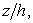the absolute magnitude of stress is augmented. In addition, for a constantthe radial stress increases for higher value of γ.

### 5. Conclusion

In this study, static analysis of three-layer functionally graded circular sandwich plates with different profiles of variable thickness, resting on Winkler elastic foundation and under uniformly distributed loads was investigated using differential quadrature method (DQM). The accuracy of the present work has been demonstrated for analysis of the simply supported and clamped plate under various parameters such as variation of the plate thickness, elastic medium coefficient, and volume fraction exponent. Some numerical examples were provided. The obtained results for the multilayer FGM circular sandwich plate using the proposed method are in accordance with FE simulations and the published works. As observed, a close agreement of the results with those available in the literatures and FE method, are achieved. A summary of the main conclusions are as follows:

Ÿ By varying the geometrical parameters of thickness profile, the amount of plate deflection is decreased for the greater value of n. In fact, when the thickness profile is linear form, the least amount of deflection occurs.

Ÿ By varying the geometrical parameters of thickness, the amount of plate displacement gets less than for the greater value of γ. In fact, when the case in which the plate profile convert to form of convex, the displacement decreases.

Ÿ By changing the FG power index of the upper and lower layers of the sandwich plate to the ceramic material, it is observed that the plate displacement is considerably reduced.

Ÿ The highest value of deflection in all cases and both supports conditions occurs in the center of the plate for foundationless condition. This point gets far from center when coefficient of elastic foundation increases. In addition, the amount of displacement in the center of simply supported plate is always more than clamped one.

Ÿ The material properties gradient influences significantly on the stress distribution across the thickness and radius as well as on the bending response of functionally graded plates under uniform loading. The flexural rigidity is decreased by increasing the volume fraction and gives rise to increase the deflection of the plate. Hence, increasing the volume fraction of FG, causes to increase the amount of the radial and circumferential stresses.

Ÿ According to the obtained results, the deflection of the plate is decreased nonlinearly by increasing the stiffness of the elastic foundation; on the other hand, by increasing the stiffness of the elastic foundation, the effect of the uniform load on the deflection is reduced. Consequently, the magnitude of the deflection and stresses can be controlled by variation of the elastic foundation stiffness and the FG power index. Moreover, it can be concluded that the plate bending is more effectively decreased by increasing the volume fraction index of the FGM circular plate on the elastic medium. The present results can be used as a benchmark to assess the validity of the results of future research in this field.

### References

  Chakraborty, A., Rahman, S., "Stochastic multiscale models for fracture analysis of functionally graded materials," Eng. Fract. Mech., 75 (8). 2062-2086. 2008. In article View Article  Koizumi, M., “the concepts of FGM, Cream Trans Funct Gradient,” Mater, 34. 3-10. 1993. In article  Miyamoto, Y., Kaysser, W.A., Rabin, B.H., Kawasaki, A., Ford, R.G., Functionally graded materials: design, processing and application, Kulwer Academic Publisher, London, 1999. In article View Article  Zenkour, A.M., "Bending analysis of functionally graded sandwich plates using a simple four-unknown shear and normal deformations theory," J Sandw Struct Mater., 15(6). 629-56. 2013. In article View Article  Nguyen, T.K., Vo, TP., Thai, H.T., “Vibration and buckling analysis of functionally graded sandwich plates with improved transverse shear stiffness based on the first-order shear deformation theory,” Proc Institution Mech Eng Part C J Mech Eng Sci., 228 (12). 2110-2131. 2014. In article View Article  Thai, H.T., Nguyen, T.K., Vo, TP., Lee, J., “Analysis of functionally graded sandwich plates using a new first-order shear deformation theory,” Eur J Mech - A/Solids, 45. 211-25. 2014. In article View Article  Thai, CH., Kulasegaram, S., Tran, LV., Nguyen-Xuan, H., “Generalized shear deformation theory for functionally graded isotropic and sandwich plates based on isogeometric approach,” Comput Struct., 141. 94-112. 2014. In article View Article  Beni, N.N., Dehkordi, M.B., “An extension of Carrera unified formulation in polar coordinate for analysis of circular sandwich plate with FGM core using GDQ method,” Composite Structures, 185. 421-434. 2018. In article View Article  Ma, L.S., Wang, T.J., “Nonlinear bending and post-buckling of a functionally graded circular plate under mechanical and thermal loadings,” Int J Solids Struct., 40. 3311-30. 2003. In article View Article  Li, D., Deng, Z., Xiao, H., Zhu, L., “Thermomechanical bending analysis of functionally graded sandwich plates with both functionally graded face sheets and functionally graded cores,” Mechanics of Advanced Materials and Structures, 25. 179-191. 2018. In article View Article  Daikh, A.A., Megueni, A., “Thermal buckling analysis of functionally graded sandwich plates,” Journal of Thermal Stresses, 41. 139-159. 2018. In article View Article  Li, D., Deng, Z., Xiao, H., Jin, P., “Bending analysis of sandwich plates with different face sheet materials and functionally graded soft core,” Thin-Walled Structures, 123. 333-340. 2018. In article View Article  Praveen, G.N., Reddy, J.N., "Nonlinear transient thermoelastic analysis of functionally graded ceramic–metal plates," Int J Solids Struct, 33. 4457-76. 1998. In article View Article  Houari, M.A., Tounsi, A., Bég, O.A., "Thermo elastic bending analysis of functionally graded sandwich plates using a new higher order shear and normal deformation theory," International Journal of Mechanical Sciences, 76. 102-111. 2013. In article View Article  Huanga, Y., Li, X.F., "Effect of radial reaction force on the bending of circular plates resting on a ring support," International Journal of Mechanical Sciences, 119. 197-207. 2016. In article View Article  Chen, J.S., Fang, Y.Y., "Non-axisymmetric warping of a heavy circular plate on a flexible ring support," Int J Solids Struct., 47. 2767-74. 2010. In article View Article  Alipour, M.M., Shariyat, M., "An elasticity-equilibrium-based zigzag theory for axisymmetric bending and stress analysis of the functionally graded circular sandwich plates, using a McLaurin-type series solution," Eur J Mech - A/Solids, 34. 78-101. 2012. In article View Article  Mantari, J.L., Monge, J.C., "Buckling, free vibration and bending analysis of functionally graded sandwich plates based on an optimized hyperbolic unified formulation," International Journal of Mechanical Sciences, 119. 170-186. 2016 In article View Article  Carrera, E., Pagani, A., Valvano, S., "Multilayered plate elements accounting for refined theories and node-dependent kinematics," Composites Part B: Engineering, 114. 189-210. 2017. In article View Article  Lokavarapu, B.R., Chellapilla, K.R., "Buckling of circular plate with foundation and elastic edge, Mechanics and Materials in Design," International Journal of Mechanics and Materials in Design, 10. p 1007. 2012. In article  Farhatnia, F., Ghanbari-Mobarakeh, M., Rasouli-Jazi, S., Oveissi, S., "Thermal buckling analysis of functionally garded circular plate resting on the Pasternak elastic foundation via differential transform," Facta Universitatis, Series: Mechanical Engineering, 15 (3). April 2017. In article View Article  Efraim, E., Eisenberger, M., "Exact vibration analysis of variable thickness thick annular isotropic and FGM plates," J Sound Vib., 299. 720-38. 2007. In article View Article  Naei, M.H., Masoumi, A., Shamekhi, A., "Buckling analysis of circular functionally graded material plate having variable thickness under uniform compression by finite-element method," Proc Inst Mech Eng Part C, J Mech Eng Sci., 221. 1241-7. 2007. In article View Article  Xiang, H.J., Yang, J., "Free and forced vibration of a laminated FGM Timoshenko beam of variable thickness under heat conduction," Compos Part B: Eng., 39. 292-303. 2008. In article View Article  Xu, Y., Zhou, D., "Three-dimensional elasticity solution of functionally graded rectangular plates with variable thickness," Compos Struct., 91. 56-65. 2009. In article View Article  Farhatnia, F., Golshah, A., "Investigation on Buckling of Orthotropic Circular and Annular Plates of Continuously Variable Thickness by Optimized Ritz Method," International Journal for Simulation and Multidisciplinary Design Optimization, (IJSMDO), 4. 127-133. 2010. In article View Article  Vivio, F., Vullo, V., "Closed form solutions of axisymmetric bending of circular plates having non-linear variable thickness," Int J Mech Sci., 52. 1234-52. 2010. In article View Article  Reddy, J.N., Theory and Analysis of Elastic Plates and Shells, Second Edition, CRC publication, 2006. In article  Szilard, R., Theories and Applications of Plate Analysis: Classical Numerical and Engineering Methods, Wiley Publisher, Germany, 2004, ISBN-13. In article View Article  Farhatnia, F., Babaei, J., Foroudastan, R., "Thermo-Mechanical Nonlinear Bending Analysis of Functionally Graded Thick Circular Plates Resting on Winkler Foundation Based on Sinusoidal Shear Deformation Theory," R. Arab J Sci Eng, 43. 1137-1151. 2018. In article View Article  Farhatnia, F., "A Theoretical Analysis of Static Response in FG Rectangular Thick Plates with a Four-Parameter Power-Law Distribution," American Journal of Mechanical Engineering, 4 (1). 11-20. 2016. In article  Poodeh, F., Farhatnia, F., Reissi, M., "Buckling analysis of orthotropic thin rectangular plates subjected to nonlinear in-plane distributed loads using generalized differential quadrature method," International Journal for Computational Methods in Engineering Science and Mechanics, 2018, 19 (2). 102-116. 2018. In article View Article  Hosseini-Hashemi, Sh., Akhavana, H., Damavandi Taherb, H.R., Daemic, N., Alibeigloo, A., "Differential quadrature analysis of functionally graded circular and annular sector plates on elastic foundation," Materials and Design, 31 (4). 1871-1880. 2010. In article View Article  Liew, K.M., Han, J.B., Xiao, Z.M., "Vibration Analysis of Circular Mindlin Plates using the Differential Quadrature Method," Journal of Sound and Vibration, 205. 617-630. 1997. In article View Article  Bert, C.W., Malik, M., "Differential Quadrature Method in Computational Mechanics: A Review," Appl. Mech. Rev, 49 (1). 1-28. 1996. In article View Article  Abbasi, S., Farhatnia, F., Jazi, S.R., "A semi-analytical solution on static analysis of circular plate exposed to non-uniform axisymmetric transverse loading resting on Winkler elastic foundation," Archives of Civil and Mechanical Engineering, 14 (3). 476-488. 2013. In article View Article

Published with license by Science and Education Publishing, Copyright © 2019 Fatemeh Farhatnia, Reza Saadat and Soheil OveissiThis work is licensed under a Creative Commons Attribution 4.0 International License. To view a copy of this license, visit http://creativecommons.org/licenses/by/4.0/

### Cite this article:

##### Normal Style
Fatemeh Farhatnia, Reza Saadat, Soheil Oveissi. Functionally Graded Sandwich Circular Plate of Non-Uniform Varying Thickness with Homogenous Core Resting on Elastic Foundation: Investigation on Bending via Differential Quadrature Method. American Journal of Mechanical Engineering. Vol. 7, No. 2, 2019, pp 68-78. http://pubs.sciepub.com/ajme/7/2/3
##### MLA Style
Farhatnia, Fatemeh, Reza Saadat, and Soheil Oveissi. "Functionally Graded Sandwich Circular Plate of Non-Uniform Varying Thickness with Homogenous Core Resting on Elastic Foundation: Investigation on Bending via Differential Quadrature Method." American Journal of Mechanical Engineering 7.2 (2019): 68-78.
##### APA Style
Farhatnia, F. , Saadat, R. , & Oveissi, S. (2019). Functionally Graded Sandwich Circular Plate of Non-Uniform Varying Thickness with Homogenous Core Resting on Elastic Foundation: Investigation on Bending via Differential Quadrature Method. American Journal of Mechanical Engineering, 7(2), 68-78.
##### Chicago Style
Farhatnia, Fatemeh, Reza Saadat, and Soheil Oveissi. "Functionally Graded Sandwich Circular Plate of Non-Uniform Varying Thickness with Homogenous Core Resting on Elastic Foundation: Investigation on Bending via Differential Quadrature Method." American Journal of Mechanical Engineering 7, no. 2 (2019): 68-78.
Share